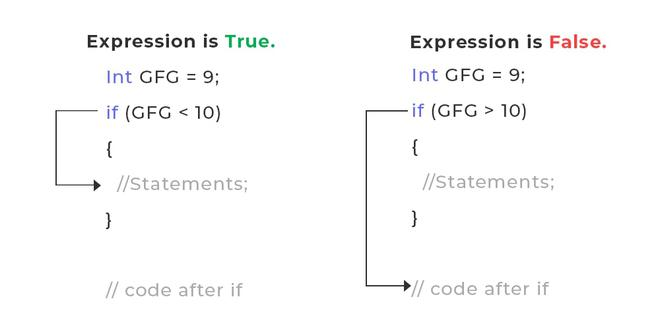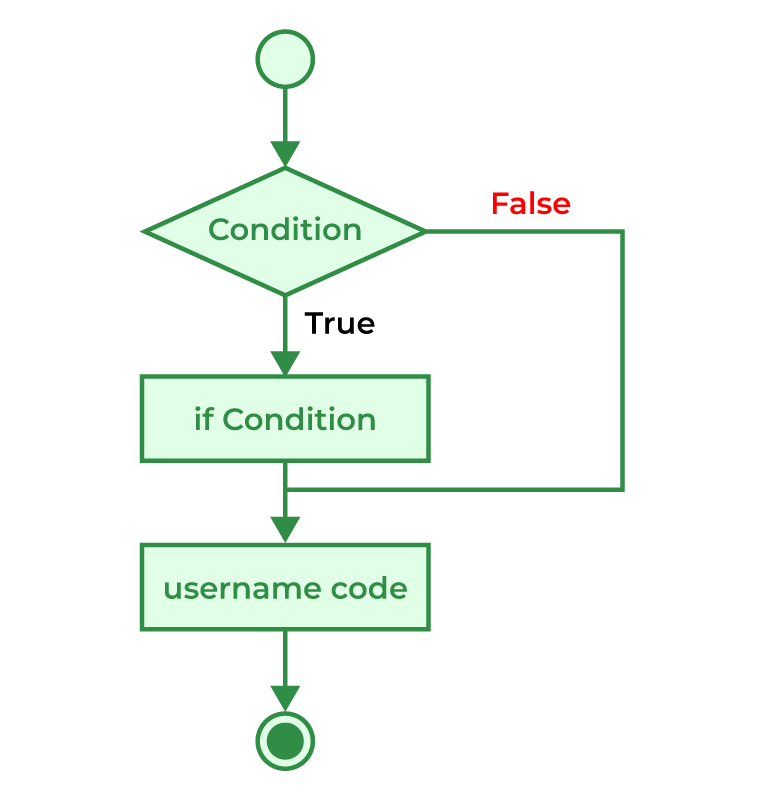Open In App
Related Articles
• Write an Interview Experience
• C Programming Language Tutorial

# C – if Statement

The if in C is the most simple decision-making statement. It consists of the test condition and if block or body. If the given condition is true only then the if block will be executed.

## What is if in C?

The if in C is a decision-making statement that is used to execute a block of code based on the value of the given expression. It is one of the core concepts of C programming and is used to include conditional code in our program.

## Syntax of if Statement in C

```if(condition)
{
// if body
// Statements to execute if condition is true
}```

## How to use if statement in C?

The following examples demonstrate how to use the if statement in C:

## C

 `// C Program to demonstrate the syntax of if statement``#include ` `int` `main()``{` `    ``int` `gfg = 9;``    ``// if statement with true condition``    ``if` `(gfg < 10) {``        ``printf``(``"%d is less than 10"``, gfg);``    ``}` `    ``// if statement with false condition``    ``if` `(gfg > 20) {``        ``printf``(``"%d is greater than 20"``, gfg);``    ``}` `    ``return` `0;``}`

Output

`9 is less than 10`

## How if in C works?Working of if Statement in C

The working of the if statement in C is as follows:

1. STEP 1: When the program control comes to the if statement, the test expression is evaluated.
2. STEP 2A: If the condition is true, the statements inside the if block are executed.
3. STEP 2B: If the expression is false, the statements inside the if body are not executed.
4. STEP 3: Program control moves out of the if block and the code after the if block is executed.

## Flowchart of if in CFlow Diagram of if Statement in C

## Examples of if Statements in C

### Example 1: C Program to find the greatest of the three numbers

In this program, we will make use of the logic that if the number is divisible by 2, then it is even else odd except one.

## C

 `// C Program to check if the number is even or odd``#include ` `int` `main()``{``    ``int` `n = 4956;` `    ``// condition to check for even number``    ``if` `(n % 2 == 0) {``        ``printf``(``"%d is Even"``, n);``        ``return` `0;``    ``}` `    ``// condition to check for odd number``    ``if` `(n % 2 != 0) {``        ``printf``(``"%d is Odd"``, n);``        ``return` `0;``    ``}``    ``return` `0;``}`

Output

`4956 is Even`

### Example 2: C Program to check whether a number is prime or not.

In this program, we will check for the smallest factor of the given number N starting from 2 to sqrt (N) using a loop. Whenever we find the factor, we will set the flag and exit the loop. The code to be executed will be contained inside the if statement.

## C

 `// C program to check whether a number is prime or not``#include ` `int` `main()``{``    ``int` `n = 19;``    ``int` `flag = 0;` `    ``for` `(``int` `i = 2; i * i <= n; i++) {` `        ``// If n is divisible by any number between``        ``// 2 and n/2, it is not prime``        ``if` `(n % i == 0) {``            ``flag = 1;``            ``break``;``        ``}``    ``}` `    ``printf``(``"%d is "``, n);``    ``if` `(flag == 1) {``        ``// it is only printed if the number is not prime``        ``printf``(``"not "``);``    ``}``    ``printf``(``"a prime number.\n"``);` `    ``return` `0;``}`

Output

`19 is a prime number.`

Following are the main advantages of the if statement in C:

• It is the simplest decision-making statement.
• It is easy to use and understand.
• It can evaluate expressions of all types such as int, char, bool, etc.

The main limitations of if block is listed below:

• It contains only a single block. In case when there are multiply related if blocks, all the blocks will be tested even when the matching if block is found at the start
• When there are a large number of expressions, the code of the if block gets complex and unreadable.
• It is slower for a large number of conditions.

## Conclusion

The if statement is the simplest decision-making statement due to which it is easy to use and understand. But being simple, it also has many limitations. We can use if-else, if-else-if ladder, or switch statements to overcome these limitations. Still, the if statement is widely used in C programming to add some conditional code to the program.

## FAQs on if in C

### 1. Define C if staement.

The if statement is a program control statement in C language that is used to execute a part of code based on some condition.

### 2. How many types of decision-making statements are there in the C language?

There are 5 types of conditional statements or decision-making statements in C language:

### 3. Can we specify multiple conditions in if statement?

We can specify multiple conditions in the if statement but not separately. We have to join these multiple conditions using logical operators making them into a single expression. We can then use this expression in the if statement.

#### Valid Expressions

```if (a < b && a < c);
if (a == 25 || a < 25);```

#### Invalid Expressions

`if (a < b, a < c);`

In the above expression, the rightmost expression in the parenthesis will be considered.